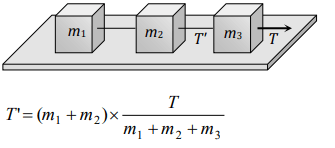## Laws of Motion Questions and Answers Part-18

1. If two forces of 5 N each are acting along X and Y axes, then the magnitude and direction of resultant is
a) $5\sqrt{2},\pi\diagup3$
b) $5\sqrt{2},\pi\diagup4$
c) $-5\sqrt{2},\pi\diagup3$
d) $-5\sqrt{2},\pi\diagup4$

Explanation:2. Which of the following is the correct order of forces
a) Weak < gravitational forces < strong forces(nuclear) < electrostatic
b) Gravitational < weak < (electrostatic) < strong force
c) Gravitational < electrostatic < weak < strong force
d) Weak < gravitational < electrostatic < strong forces

Explanation: Gravitational < weak < (electrostatic) < strong force

3. A block is kept on a frictionless inclined surface with angle of inclination $'\alpha'$. The incline is given an acceleration 'a' to keep the block stationary. Then a is equal to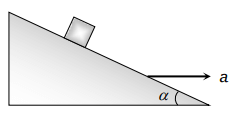a) g
b) g$tan\alpha$
c) g$\diagup tan\alpha$
d) g$cosec \alpha$

Explanation:4. A block of mass M is pulled along a horizontal frictionless surface by a rope of mass m. If a force P is applied at the free end of the rope, the force exerted by the rope on the block will be
a) P
b) $\frac{Pm}{M+m}$
c) $\frac{PM}{M+m}$
d) $\frac{Pm}{M-m}$

Explanation:5. A rope of length L is pulled by a constant force F. What is the tension in the rope at a distance x from the end where the force is applied
a) $\frac{FL}{x}$
b) $\frac{F\left(L-x\right)}{L}$
c) $\frac{FL}{L-x}$
d) $\frac{Fx}{L-x}$

Explanation: $\frac{F\left(L-x\right)}{L}$

6. Three equal weights A, B and C of mass 2 kg each are hanging on a string passing over a fixed frictionless pulley as shown in the figure The tension in the string connecting weights B and C is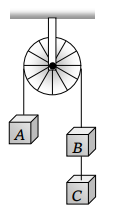a) Zero
b) 13 N
c) 3.3 N
d) 19.6 N

Explanation: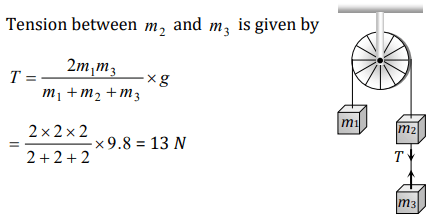7. Two masses 2 kg and 3 kg are attached to the end of the string passed over a pulley fixed at the top. The tension and acceleration are
a) $\frac{7g}{8};\frac{g}{8}$
b) $\frac{21g}{8};\frac{g}{8}$
c) $\frac{21g}{8};\frac{g}{5}$
d) $\frac{12g}{5};\frac{g}{5}$

Explanation: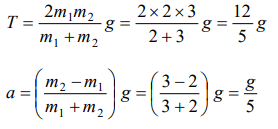8. Three blocks A, B and C weighing 1, 8 and 27 kg respectively are connected as shown in the figurewith an inextensible string and are moving on a smooth surface. $T_{3}$ is equal to 36 N. Then $T_{2}$ isa) 18 N
b) 9 N
c) 3.375 N
d) 1.25 N

Explanation: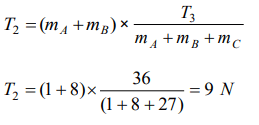9. Two bodies of mass 3 kg and 4 kg are suspended at the ends of massless string passing over a frictionless pulley. The acceleration of the system is $\left(g=9.8m\diagup s^{2}\right)$
a) $4.9m\diagup s^{2}$
b) $2.45m\diagup s^{2}$
c) $1.4m\diagup s^{2}$
d) $9.5m\diagup s^{2}$10. Three solids of masses $m_{1},m_{2}$  and $m_{3}$  are connected with weightless string in succession and are placed on a frictionless table. If the mass $m_{3}$ is dragged with a force T, the tension in the string between $m_{2}$  and $m_{3}$  is
a) $\frac{m_{2}}{m_{1}+m_{2} +m_{3}}T$
b) $\frac{m_{3}}{m_{1}+m_{2} +m_{3}}T$
c) $\frac{m_{1}+m_{2}}{m_{1}+m_{2} +m_{3}}T$
d) $\frac{m_{2}+m_{3}}{m_{1}+m_{2} +m_{3}}T$